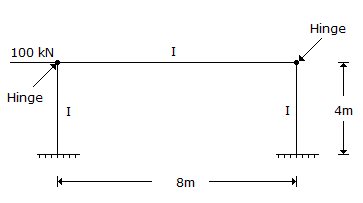# Civil Engineering - GATE Exam Questions - Discussion

### Discussion :: GATE Exam Questions - Section 1 (Q.No.40)

40.

For the frame shown in the below figure, the maximum bending moment in the column is,[A]. zero [B]. 400 kNm [C]. 100 kNm [D]. 200 kNm

Explanation:

No answer description available for this question.

 Navaneetha said: (Apr 21, 2014) There is no bending moment at hinge supports so here bending moment exist at fixed supports. Given frame is symmetrical about vertical axis. The bending moment is same at two fixed supports and which is equal to M=100*4. =400 kNm.

 Sai said: (May 25, 2015) Think it as cantilever max b.m is 100X4 = 400 KN.

 Nayan said: (Jun 4, 2015) There is no external load on beam and at hinge support BM will be zero. BM will be exist at fixed support only. 100*4 = 400 KN.

 Snehal Wankhede said: (Aug 31, 2016) I think option d) 200kNm. # according to Sai, considering it as cantilever will entire 100kn is taken by left column? As he suggested. Here is my explanation. 1) 100kn generate sway of δ which generates moment - 6EIδ/l2 2) As there is hinge at pt B/C + 6EIδ/l2 will generate to make total moment at B/C =0 3) Half of that moment is transfered to pt A/D, total moment at A/D= -6 +3=-3EIδ/l2 4) This moment generate reaction at B/C 3EIδ/l3 in +x direction = total reaction 6EIδl/l3=100 5) M= 3EIδ/l2 = 100x4/2 = 200.

 Bala said: (Dec 25, 2016) Easy way to understand this question is we know that bending moment unit KNm. In that question load is 100kN. Then multiple with some given metre value in that question 100 * 4 = 400KNm.

 Vaibhav said: (Jul 29, 2017) @Snehal probably your logic holds right. We generally solve by zeroing the moment at a hinge and transferring half the opposite applied at a hinge on the fixed side. So, (3EI(δ)/(L*L*L)) + ((3EI(δ)/(L*L*L))= 100. and Moment = (6EI(δ)/L*L) = 200.

 Satyam said: (Feb 6, 2018) On each support 50 kn horizontal force will act. So resultant force in one column=100-50=50 so BM=50*4= 200. Correct answer should be (d).

 Basu said: (Jul 25, 2018) 200 kNm is the right answer.